Tamil Nadu Board of Secondary EducationSSLC (English Medium) Class 6th

# Tamil Nadu Board Samacheer Kalvi solutions for Class 6th Mathematics Term 3 Answers Guide chapter 3 - Perimeter and Area [Latest edition]

#### Chapters## Chapter 3: Perimeter and Area

Exercise 3.1Exercise 3.2
Exercise 3.1 [Pages 51 - 53]

### Tamil Nadu Board Samacheer Kalvi solutions for Class 6th Mathematics Term 3 Answers Guide Chapter 3 Perimeter and AreaExercise 3.1 [Pages 51 - 53]

Exercise 3.1 | Q 1. i) | Page 51

The table given below contains some measures of the rectangle. Find the unknown values.

 Length Breadth Perimeter Area 5 cm 8 cm ? ?
Exercise 3.1 | Q 1. ii) | Page 51

The table given below contains some measures of the rectangle. Find the unknown values.

 Length Breadth Perimeter Area 13 cm ? 54 cm ?
Exercise 3.1 | Q 1. iii) | Page 51

The table given below contains some measures of the rectangle. Find the unknown values.

 Length Breadth Perimeter Area ? 15 cm 60 cm ?
Exercise 3.1 | Q 1. iv) | Page 51

The table given below contains some measures of the rectangle. Find the unknown values.

 Length Breadth Perimeter Area 10 m ? ? 120 sq.m
Exercise 3.1 | Q 1. v) | Page 51

The table given below contains some measures of the rectangle. Find the unknown values.

 Length Breadth Perimeter Area ? 4 feet ? 20 sq.feet
Exercise 3.1 | Q 2. i) | Page 51

The table given below contains some measures of the square. Find the unknown values.

 Side Perimeter Area 6 cm ? ?
Exercise 3.1 | Q 2. ii) | Page 51

The table given below contains some measures of the square. Find the unknown values.

 Side Perimeter Area ? 100 m ?
Exercise 3.1 | Q 2. iii) | Page 51

The table given below contains some measures of the square. Find the unknown values.

 Side Perimeter Area ? ? 49 sq.feet
Exercise 3.1 | Q 3. i) | Page 51

The table given below contains some measures of the right angled triangle. Find the unknown values.

 Base Height Area 20 cm 40 cm ?
Exercise 3.1 | Q 3. ii) | Page 51

The table given below contains some measures of the right angled triangle. Find the unknown values.

 Base Height Area 5 feet ? 20 sq.feet
Exercise 3.1 | Q 3. iii) | Page 51

The table given below contains some measures of the right angled triangle. Find the unknown values.

 Base Height Area ? 12 m 24 sq.m
Exercise 3.1 | Q 4. i) | Page 51

The table given below contains some measures of the triangle. Find the unknown values.

 Side 1 Side 2 Side 3 Perimeter 6 cm 5 cm 2 cm ?
Exercise 3.1 | Q 4. ii) | Page 51

The table given below contains some measures of the triangle. Find the unknown values.

 Side 1 Side 2 Side 3 Perimeter ? 8 m 3 m 17 m
Exercise 3.1 | Q 4. iii) | Page 51

The table given below contains some measures of the triangle. Find the unknown values.

 Side 1 Side 2 Side 3 Perimeter 11 feet ? 9 feet 28 feet

#### Fill in the blanks

Exercise 3.1 | Q 5. i) | Page 51

5 cm2 = _______ mm2

Exercise 3.1 | Q 5. ii) | Page 51

26 m2 = _______ cm2

Exercise 3.1 | Q 5. iii) | Page 51

8 km2 = _______ m2

Exercise 3.1 | Q 6. i) | Page 52

Find the perimeter and area of the following shape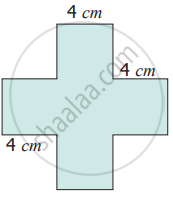Exercise 3.1 | Q 6. ii) | Page 52

Find the perimeter and area of the following shape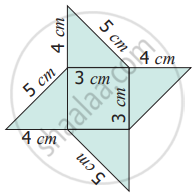Exercise 3.1 | Q 6. iii) | Page 52

Find the perimeter and area of the following shape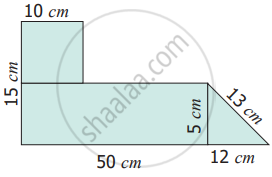Exercise 3.1 | Q 7 | Page 52

Find the perimeter and the area of the rectangle whose length is 6 m and breadth is 4 m

Exercise 3.1 | Q 8 | Page 52

Find the perimeter and the area of the square whose side is 8 cm

Exercise 3.1 | Q 9 | Page 52

Find the perimeter and the area of a right angled triangle whose sides are 6 feet, 8 feet and 10 feet

Exercise 3.1 | Q 10. i) | Page 52

Find the perimeter of A scalene triangle with sides 7 m, 8 m, 10 m

Exercise 3.1 | Q 10. ii) | Page 52

Find the perimeter of An isosceles triangle with equal sides 10 cm each and third side is 7 cm

Exercise 3.1 | Q 10. iii) | Page 52

Find the perimeter of An equilateral triangle with side 6 cm

Exercise 3.1 | Q 11 | Page 52

The area of a rectangular shaped photo is 820 sq.cm. and its width is 20 cm. What is its length? Also find its perimeter

Exercise 3.1 | Q 12 | Page 52

A square park has 40 m as its perimeter. What is the length of its side? Also find its area

Exercise 3.1 | Q 13 | Page 52

The scalene triangle has 40 cm as its perimeter and whose two sides are 13 cm and 15 cm, find the third side

Exercise 3.1 | Q 14 | Page 52

A field is in the shape of a right angled triangle whose base is 25 m and height 20 m. Find the cost of levelling the field at the rate of ₹ 45 per sq.m2

Exercise 3.1 | Q 15 | Page 52

A square of side 2 cm is joined with a rectangle of length 15 cm and breadth 10 cm. Find the perimeter of the combined shape

#### Objective Type Questions

Exercise 3.1 | Q 16 | Page 52

The following figure are of equal area. Which figure has the least perimeter?

•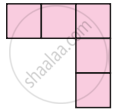•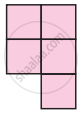•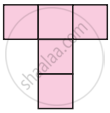•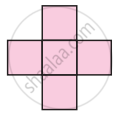Exercise 3.1 | Q 17 | Page 53

If two identical rectangles of perimeter 30 cm are joined together, then the perimeter of the new shape will be

• equal to 60 cm

• less than 60 cm

• greater than 60 cm

• equal to 45 cm

Exercise 3.1 | Q 18 | Page 53

If every side of a rectangle is doubled, then its area becomes _______ times

• 2

• 3

• 4

• 6

Exercise 3.1 | Q 19 | Page 53

The side of a square is 10 cm. If its side is tripled, then by how many times will its perimeter increase?

• 2 times

• 4 times

• 6 times

• 3 times

Exercise 3.1 | Q 20 | Page 53

The length and breadth of a rectangular sheet of a paper are 15 cm and 12 cm respectively. A rectangular piece is cut from one of its corners. Which of the following statement is correct for the remaining sheet?

• Perimeter remains the same but the area changes

• Area remains the same but the perimeter changes

• There will be a change in both area and perimeter.

• Both the area and perimeter remains the same.

Exercise 3.2 [Pages 53 - 54]

### Tamil Nadu Board Samacheer Kalvi solutions for Class 6th Mathematics Term 3 Answers Guide Chapter 3 Perimeter and AreaExercise 3.2 [Pages 53 - 54]

#### Miscellaneous Practice Problems

Exercise 3.2 | Q 1. i) | Page 53

A piece of wire is 36 cm long. What will be the length of each side if we form a square

Exercise 3.2 | Q 1. ii) | Page 53

A piece of wire is 36 cm long. What will be the length of each side if we form an equilateral triangle

Exercise 3.2 | Q 2 | Page 53

From one vertex of an equilateral triangle with side 40 cm, an equilateral triangle with 6 cm side is removed. What is the perimeter of the remaining portion?

Exercise 3.2 | Q 3 | Page 53

Rahim and Peter go for a morning walk, Rahim walks around a square path of side 50 m and Peter walks around a rectangular path with length 40 m and breadth 30 m. If both of them walk 2 rounds each, who covers more distance and by how much?

Exercise 3.2 | Q 4 | Page 53

The length of a rectangular park is 14 m more than its breadth. If the perimeter of the park is 200 m, what is its length? Find the area of the park

Exercise 3.2 | Q 5 | Page 53

Your garden is in the shape of a square of side 5 m. Each side is to be fenced with 2 rows of wire. Find how much amount is needed to fence the garden at ₹ 10 per metre

#### Challenge Problems

Exercise 3.2 | Q 6 | Page 53

A closed shape has 20 equal sides and one of its sides is 3 cm. Find its perimeter

Exercise 3.2 | Q 7 | Page 53

A rectangle has length 40 cm and breadth 20 cm. How many squares with side 10 cm can be formed from it

Exercise 3.2 | Q 8 | Page 53

The length of a rectangle is three times its breadth. If its perimeter is 64 cm, find the sides of the rectangle

Exercise 3.2 | Q 9 | Page 54

How many different rectangles can be made with a 48 cm long string? Find the possible pairs of length and breadth of the rectangles.

Exercise 3.2 | Q 10 | Page 54

Draw a square B whose side is twice of the square A. Calculate the perimeter of the squares A and B

Exercise 3.2 | Q 11 | Page 54

What will be the area of a new square formed if the side of a square is made one-fourth?

Exercise 3.2 | Q 12 | Page 54

Two plots have the same perimeter. One is a square of side 10 m and another is a rectangle of breadth 8 m. Which plot has the greater area and by how much?

Exercise 3.2 | Q 13 | Page 54

Look at the picture of the house given and find the total area of the shaded portion.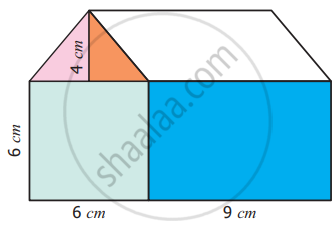Exercise 3.2 | Q 14 | Page 54

Find the approximate area of the flower in the given square grid.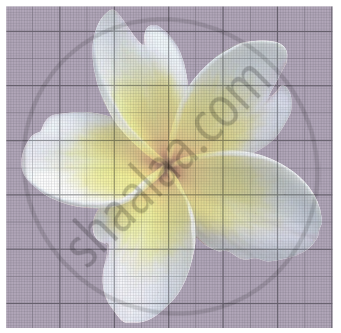## Chapter 3: Perimeter and Area

Exercise 3.1Exercise 3.2## Tamil Nadu Board Samacheer Kalvi solutions for Class 6th Mathematics Term 3 Answers Guide chapter 3 - Perimeter and Area

Tamil Nadu Board Samacheer Kalvi solutions for Class 6th Mathematics Term 3 Answers Guide chapter 3 (Perimeter and Area) include all questions with solution and detail explanation. This will clear students doubts about any question and improve application skills while preparing for board exams. The detailed, step-by-step solutions will help you understand the concepts better and clear your confusions, if any. Shaalaa.com has the Tamil Nadu Board of Secondary Education Class 6th Mathematics Term 3 Answers Guide solutions in a manner that help students grasp basic concepts better and faster.

Further, we at Shaalaa.com provide such solutions so that students can prepare for written exams. Tamil Nadu Board Samacheer Kalvi textbook solutions can be a core help for self-study and acts as a perfect self-help guidance for students.

Concepts covered in Class 6th Mathematics Term 3 Answers Guide chapter 3 Perimeter and Area are Concept of Perimeter, Perimeter of a Rectangle, Perimeter of Squares, Perimeter of Triangles, Concept of Area, Area of Rectangle, Area of Square, Area of a Triangle, Perimeter and Area of Combined Shapes, Estimate the Area of Irregular Shape Using a Graph Paper.

Using Tamil Nadu Board Samacheer Kalvi Class 6th solutions Perimeter and Area exercise by students are an easy way to prepare for the exams, as they involve solutions arranged chapter-wise also page wise. The questions involved in Tamil Nadu Board Samacheer Kalvi Solutions are important questions that can be asked in the final exam. Maximum students of Tamil Nadu Board of Secondary Education Class 6th prefer Tamil Nadu Board Samacheer Kalvi Textbook Solutions to score more in exam.

Get the free view of chapter 3 Perimeter and Area Class 6th extra questions for Class 6th Mathematics Term 3 Answers Guide and can use Shaalaa.com to keep it handy for your exam preparation